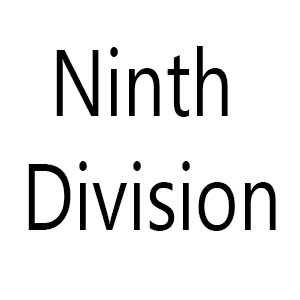Navamsa ( Ninth Division )

Navamsa chart is a very important sub-division of natal chart in vedic astrology. Navamsa chart is said as fruit of natal chart. Almost every astrologer likes to look Navamsa chart with natal Chart.

Ninth division of a rasi is known as a Navamsa.

1 Navamsa = 300/9 = 3020’We have to take degree of a planet and divide it by 3020’, If the answer is in decimal, we have to round up the answer.

1)     If the planet is in Movable sign in natal Chart, we will start counting from the sign where planet is situated up to the number which we have found from the division of planet degree by 3020’, In navamsa chart we will put the planet where the counting ends.

2)     If the planet is in Fixed sign in natal Chart, we will start counting from the 9th sign where planet is situated up to the number which we have found from the division of planet degree by 3020’, In navamsa chart we will put the planet where the counting ends.

3)     If the planet is in Dual sign in natal Chart, we will start counting from the 5th sign where planet is situated up to the number which we have found from the division of planet degree by 3020’, In navamsa chart we will put the planet where the counting ends.

In natal Chart Ascendant ( Lagna ) is in Mesh ( Aries ) at 3023’

So according to above mentioned rule we have to divide ascendant degree by 3020’

= 3023’/3020’ = 1+ then we will round up the number that is = 2

Because Mesh ( Aries ) is a movable sign we will start counting from Mesh ( Aries ) to 2nd  houses, so we will find vrisha ( Taurus ) that means in navamsa Chart Ascendant will fall in Vrisha Rashi ( Taurus )

Let’s take Rahu which is in Kanya ( Virgo ) at 9053’

So, we divide the Rahu degree by 3020’

= 9053’ / 3020’ = 2 + then we will round up the number that is = 3

Virgo is a Dual Movement sign so we will count 3rd from the 5th of Virgo which is Meena ( Pisces ). Rahu will fall in Meen ( Pisces ) in Navamsa Chart.

Let’s take Jupiter ( Guru ) which is in Kumbha ( Aquarius ) at 23046’

So, we divide Jupiter degree by 3020’

= 23046’ / 3020’ = 7+ then we will round up the number that is = 8

Kumbha is a Fixed Sign so we will count 8th from the 9th of Kumbha which is vrisha ( Taurus ). Jupiter will fall in Vrisha ( Taurus ).